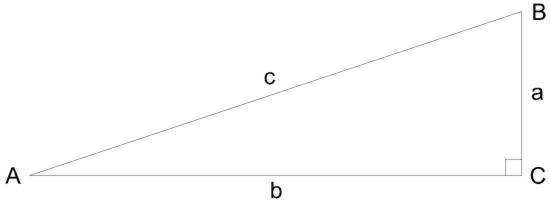The Sundial Primer created by Carl SabanskiThe Sundial Primer Index
 Trigonometry Trigonometry: is the branch of mathematics that treats of the relations of the sides and angles of triangles and of the methods of applying these relations in the solution of problems involving triangles. (Webster) Trigonometry is all about triangles! Trigonometry has a major role in the design of sundials. The following is a little information on some of the trig functions you will discover. Perhaps it may help. Angle An angle of one degree is an angle in which the rotation is 1/360 of one complete rotation. A straight angle is an angle of 180° (180 degrees). A right angle is an angle of 90°. An acute angle is an angle between 0° and 90°. An obtuse angle is an angle between 90° and 180°. A radian is an angle subtended at the centre of a circle by and arc whose angle is equal to that of the radius. 180° = π radians; 1° = π / 180 radians; 1 radian = 180 / π degrees The Trigonometric Functions of an Acute AngleIn a right triangle ABC: sine A = sin A = a / c cosine A = cos A = b / c tangent A = tan A = a / b c2 = a2 + b2